Sequence

The head Sequence is rarely seen in directly.  Consider the function (f)
below, where the first two arguments are p1,and p2, and all the remaining
arguments are part of p3.  All the remaining arguments go with p3 because the
definition of (f) uses BlankSequence (___) for p3.  The only thing the
function (f) does is return p3.  One might wonder what head is given to p3.
It turns out it is given the head Sequence. After (f) is defined, we use it
to make the Sequence (seq).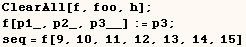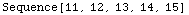If you have a number of sequences inside almost any head the sequences are  automatically flattened, and the fact that the arguments are a sequence is  not indicated (see the example in the next line).  The only functions that  don't splice the sequences together are those with the Attribute SequenceHold.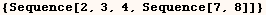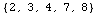Most useful applications of Sequence are variations of the next cell where
the head (h) is changed to Sequence and the effect is that h[7,11,13] is
changed to (7,11,13).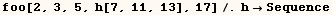Created by Mathematica  (May 16, 2004)

Back to Ted’s Tricks index page